Chord (aircraft)By Wikipedia,
the free encyclopedia,

https://en.wikipedia.org/wiki/Chord_(aeronautics)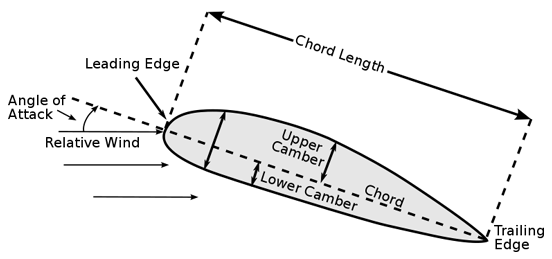Cross section of an airfoil showing chord and chord length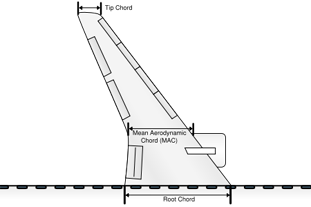The various chords on the planform of the swept-wing of an aircraft

In aeronauticschord refers to the imaginary straight line joining the trailing edge and the center of curvature of the leading edge of the cross-section of an airfoil. The chord length is the distance between the trailing edge and the point on the leading edge where the chord intersects the leading edge. The wing, horizontal stabilizer, vertical stabilizer and propeller of an aircraft are all based on airfoil sections, and the term chord or chord length is also used to describe their width. The chord of a wing, stabilizer and propeller is determined by examining the planform and measuring the distance between leading and trailing edges in the direction of the airflow. (If a wing has a rectangular planform, rather than tapered or swept, then the chord is simply the width of the wing measured in the direction of airflow.) The term chord is also applied to the width of wing flaps, ailerons and rudder on an aircraft. Most wings do not have a rectangular planform so they have a different chord at different positions along their span. To give a characteristic figure which can be compared among various wing shapes, the mean aerodynamic chord, or MAC, is used. The MAC is somewhat more complex to calculate, because most wings vary in chord over the span, growing narrower towards the outer tips. This means that more lift is generated on the wider inner portions, and the MAC moves the point to measure the chord to take this into account.

## Standard mean chord

Standard mean chord (SMC) is defined as wing area divided by wing span: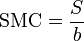$\mbox{SMC} = \frac{S}{b}$,

where S is the wing area and b is the span of the wing. Thus, the SMC is the chord of a rectangular wing with the same area and span as those of the given wing. This is a purely geometric figure and is rarely used in aerodynamics.

## Mean aerodynamic chord

Mean aerodynamic chord (MAC) is defined as: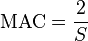$\mbox{MAC} = \frac{2}{S}$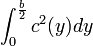$\int_{0}^{\frac{b}{2}}c^2(y) dy$ ,

where y is the coordinate along the wing span and c(y) is the chord at the coordinate y. Other terms are as for SMC. Physically, MAC is the chord of a rectangular wing, which has the same area, aerodynamic force and position of the center of pressure at a given angle of attack as the given wing has. Simply stated, MAC is the width of an equivalent rectangular wing in given conditions. Therefore, not only the measure but also the position of MAC is often important. In particular, the position of center of mass (CoM) of an aircraft is usually measured relative to the MAC, as the percentage of the distance from the leading edge of MAC to CoM with respect to MAC itself. Note that the figure to the right implies that the MAC occurs at a point where leading or trailing edge sweep changes. In general, this is not the case. Any shape other than a simple trapezoid requires evaluation of the above integral. The ratio of the length (or span) of a wing to its chord is known as the aspect ratio, an important indicator of the lift-induced drag the wing will create. In general, planes with higher aspect ratios — long, skinny wings — will have less induced drag, which dominates at low airspeeds. This is why gliders have long wings.

## Tapered wing

Knowing the area (Sw), taper ratio (λ) and the span (b) of the wing, and whether the wing has sweep or not, the chord at any position on the span can be calculated by the formula: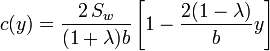$c(y)=\frac{2\,S_w}{(1+\lambda)b}\left[1-\frac{2(1-\lambda)}{b}y\right]$

Text from Wikipedia is available under the Creative Commons Attribution/Share-Alike License; additional terms may apply.

Published - July 2009

Please see some ads intermixed with other content from this site:Copyright 2004-2021 © by Airports-Worldwide.com, Vyshenskoho st. 36, Lviv 79010, Ukraine
Legal Disclaimer